# Superlattice Growth via MBE and Green’s Function Techniques

## Abstract

A model has been developed to simulate the growth of arrays consisting of a substrate on which alternating layers of quantum dots (QDs) and spacer layers are epitaxially grown. The substrate and spacer layers are modeled as an anisotropic elastic half-space, and the QDs are modeled as point inclusions buried within the half-space. In this model, the strain at the free surface of this half-space due to the buried point QDs is calculated, and a scalar measure of the strain at the surface is subsequently determined. New point QDs are placed on the surface where the previously calculated scalar strain measure is a minimum. Following available DFT results, this scalar strain measure is a weighted average of the in-plane strains. This model is constructed under the assumption that diffusional anisotropy can be neglected, and thus, the results are more in agreement with results from experiments of growth of SiGe QDs than experiments involving QDs of (In,Ga)As.

## Introduction

Arrays of quantum dots (QDs) show promise in optoelectronic applications, such as semiconductor lasers [1, 2] and photodetectors . A layer of semiconductor QDs can be grown through Stranski-Krastanov (S-K) growth , where a wetting layer of one material, such as Ge or InAs, is epitaxially grown on top of a layer of another material with a different lattice constant, such as Si or GaAs. The strain from the lattice mismatch is partially relieved through the formation of three-dimensional mounds in the wetting layer, and these mounds can then be used as quantum dots. A three-dimensional array of QDs can be formed from multiple layers of QDs, as illustrated in Fig. 1. For example, InAs QDs can be formed from alternating thick layers of GaAs and thin layers of InAs, where S-K growth occurs in the thin layers .

During epitaxy, adatoms become adsorbed onto binding sites on the substrate and then diffuse, hopping from one binding site to another with a rate of diffusion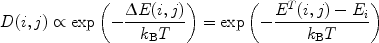(1)

where T is the temperature, k B is the Boltzmann constant,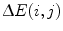is the energy barrier to diffusion, and i and j are the labels of the binding sites at the beginning and end of an adatom’s hop. E T(i, j) is the energy at the saddle point between the energy minima at sites i and j, and E i is the local energy minimum at site i. If the zero energy datum for E T(i, j) and E i is set appropriately, then E i is the binding or adsorption energy as well and is a measure of the reduction in total energy due to the adatom binding to the substrate [7, 8]. The reconstruction of semiconductor surfaces to minimize the number of dangling bonds  may cause different kinds of binding sites on the surface, e.g. one site may be between the two atoms of a surface dimer, while another is within a trench between two dimers . The energy barrieris influenced by, among other things, the strain field in the underlying substrate [7, 11]. According to ab initio simulations, for an isolated adatom on a (001) semiconductor surface, the dependence ofon strain is roughly linear for small strains [7, 1113]. In particular, the work of Shu et al.  specifies the following dependence on strain.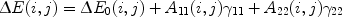(2)

γ11 and γ22 are components of the strain in the substrate (which is taken to be homogeneous here) along the - and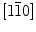-directions, and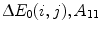, and A 22 are constants. Usually on semiconductor surfaces,tends to increase with increasing compressive strain and decrease with increasing tensile strain. However, there are exceptions to this, since the constants A 11 and A 22 can be positive, and for sufficiently large strains, the linear dependence breaks down. For example, Penev et al.  have found that for one binding site on a GaAs(001)-c(4 × 4) surface, the energy barrier for an adatom could reach a local maximum for a substrate strain of γ11 = γ22 ≈ −0.04. However, both the saddle point and binding energies corresponding to this energy barrier increase with increasing compressive strain and decrease with increasing tensile strain and do not peak for −4% strain. (The binding energy is negative, so a decrease in it corresponds to an increase in its magnitude.)

The dependence of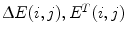, and E i on strain is indirectly taken into account in certain models of the growth of arrays of QDs where a scalar measure of the strain due to buried quantum dots, SM(x), is taken for points x at the surface, and QDs are then placed at minima of SM(x). The choice of SM(x) implies a choice of certain assumptions about this dependence on strain. For example, in the idealized two-dimensional model of the growth of QD arrays by Tersoff et al. , QDs buried within a isotropic elastic half-space (or half-plane, to be precise, since the model is 2D) cause patterns of strain minima and maxima at the surface of the half-space, and new QDs at the surface are placed at the strain minima. In that 2D model, the surface strain is a scalar, and the QDs are treated as points with SM(x) being just the strain. A similar model was developed by Liu et al. . Like the model of Tersoff et al., it is two-dimensional, but it takes into account the width of islands rather than treating them as points. In both models, tensile strain is taken to be negative, opposite of the usual engineering convention . On the one hand, this makes sense experimentally, since QDs tend to nucleate above buried QDs, and tensile strain is generally higher above the buried dots. Furthermore, as pointed out by Penev et al. , the magnitude of the binding energy increases with increasing tensile strain, so the total energy of the system is reduced by adatoms congregating near where the tensile strain is maximum. On the other hand, if one assumes that the strain in the part of the capping layer surface above a buried QD is simply higher than that of other regions of the capping layer surface, as both Tersoff et al.  and Liu et al.  appear to do, then the choice of SM(x) also implies that the energy barrier to diffusion on strain is expected to increase with increasing tensile strain as well, since otherwise, adatoms would be more prone to diffuse in, and thus move away from, the regions of higher tensile strain. Such a dependence of the diffusion barrier on strain, though, is in contrast to the findings of the ab initio simulations mentioned above. Another example is the three-dimensional model of Holý et al. , which takes into account the anisotropy of the substrate but treats the QDs as points. New QDs tend to form where the normalized strain energy density at the surface,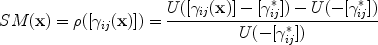(3)

is a local minimum. The sign convention for strains here—and for the rest of this paper—is the usual one. Negative is compressive, and positive is tensile . Here, [γ ij (x)] is the strain tensor at point x at the surface of the QD array before a new wetting layer is deposited. The strain energy density U([γ ij ]) equals C ijkl γ ij γ kl , where C ijkl is the elasticity tensor, and the usual Einstein summation convention is used.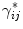is the lattice mismatch strain and equals δ ij (a QDa matrix)/a matrix, where δ ij is the Kronecker delta, and a QD and a matrix are the lattice constants of the material of the QD (e.g. InAs) and the surrounding matrix (e.g. GaAs), respectively. The second term of the sum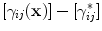in Eq. 3 takes into account the strain due to wetting layer itself, which is the opposite of the lattice mismatch strain. This model, unlike that of Tersoff et al. , is not entirely deterministic, since the locations of the QDs in each layer are determined by randomly depositing adatoms onto a layer and letting them diffuse toward the nearest minima of ρ. Figure 2 shows a profile of ρ([γ ij (x)]) along a line in the -direction passing through x = (0, 0, 0) due to a InAs QD buried in GaAs at (0, 0, 50 nm) with an effective volume equal to the volume of a pyramid with 20 and 50 nm base length (same as the effective volume used in Ref. ). The figure also shows the corresponding profile of the average in-plane strain, (γ11 + γ22)/2, due to the same QD, and this profile looks nearly like an upside-down version of the profile of the normalized strain energy, which indicates that the model of Holý et al.  in effect implies that the energy barrier to diffusion increases with increasing tensile strain.

In this paper, we take an approach similar to that of Tersoff et al.  and of Holý et al. , but seek to make the physical justification of our choice of SM(x) more clear and to explore the extent to which our approach can possibly reproduce experimental results. The connection between the diffusion barrier and SM(x) will be made explicit, and the drawbacks of using a scalar measure SM(x) to determine the locations of quantum dots will be discussed as well.

## Modeling Approach

Figure 1 schematically shows layers of irregularly arranged point QDs separated by GaAs spacer layers of thickness h and covered by a GaAs capping layer, which is also of thickness h. An imaginary grid is shown superimposed on the surface, along with a set of coordinate axes. To determine the locations of the QDs that will be deposited on the surface of the capping layer, we calculate the strain γ kl (x) at each point x in the grid as follows.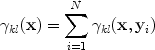(4)

The strain tensor γ kl (x, y i ) is the contribution to the strain at x from the QD at position y i , and N is the total number of QDs in all the layers within the simulation domain. This strain tensor γ kl (x, y i ) is calculated according to the methodology described by Pan [18, 19], where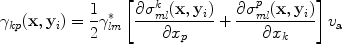(5)

The tensor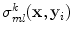is the Green’s function for the stress in an anisotropic elastic half-space .is the misfit strain tensor, whose only nonzero elements are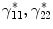, and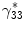. For semiconductors with the zincblende crystal structure, these nonzero elements all have the same value, i.e. the misfit strain between the substrate and the QD. v a is the effective volume of the point QD. Accordingly, the effective radius of the QD is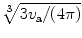.

Periodic boundary conditions are imposed along the x 1- and x 2-directions, which are along the  anddirections. QDs whose depth below the surface is greater than 120 nm are not counted in the above summation in Eq. 4, since at this depth, these QDs have little effect on the surface, as can be seen in Table 1. So that the result from these simulation can be compared with those from one of the authors [5, 20, 21], the QDs here are taken to be In0.4Ga0.6As, and accordingly, the misfit strain in each dot is taken to be 3% rather than the 7% it would be if the QDs were pure InAs. The effective radius of each of these point QDs is taken to be 3.5 nm, half the expected height of the QDs as determined from the results of Schmidbauer et al. . The grid is 200 × 200, and the dimensions of the domain in the x 1- and x 2-directions are 960 nm × 960 nm. The growth simulations are done for h equaling 14, 18, 24, 28, or 32 nm. In the initial layer, the QDs are arranged in grids of 10 × 10 and 10 × 20 but with the locations of the QDs randomly perturbed from their grid locations, as shown in Fig. 3. Once the strain at each point is determined, the following scalar measure SM(x) of the strain is calculated,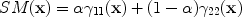(6)

The weighting factor α is taken to be a nonnegative fraction. The QDs are placed at grid points where SM(x) is the lowest local minimum. Once the new QDs are placed, the spacer thickness h is added to the x 3-coordinates of all the QDs, simulating the growth of a capping layer of GaAs with thickness h on top of the newly grown QDs. Then, we calculate SM(x) again to determine the locations of the QDs in the next layer, and so on.

The physical justifications for our choice of SM(x) in Eq. 6 is as follows. We suppose that there exists a mean energy barrier,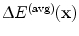, and further, that this barrier has the same kind of dependence on strain as the energy barriersin Eq. 2.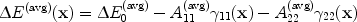(7)

Here, it is assumed, following Refs. [7, 1113] thatincreases with increasing compressive strain and decreases with increasing tensile strain, so the signs before the second and third terms on the right-hand side of Eq. 7 are negative while the coefficientsand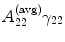are taken as positive. The above equation can be rewritten as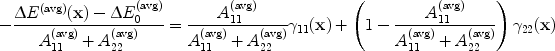(8)

which is equivalent to Eq. 6 when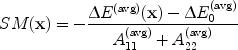(9)(10)

Atoms then are more likely to congregate where diffusion is slower, that is, where SM(x) is minimum, since SM(x) decreases as the energy barriers to diffusion increase.

There are two key assumptions involved in the formulation of SM(x). The first assumption, of course, is that the energy barriers to diffusion generally decrease as γ11(x) and γ22(x) increase. The second assumption is that there is a mean barrier to diffusionto begin with. This is essentially the same as assuming that there is a single value that governs the escape of an adatom from its current binding site, an assumption that is violated, for example, if because of surface reconstruction, the barrier for an adatom to escape along the-direction is lower than that for an adatom to escape along the -direction. The latter assumption, however, is inherent in any formulation of SM(x), since such a formulation amounts to using a single scalar value as a proxy measure of the energy barrier to diffusion.

## Results and Discussion

The calculated strains at the surface of the GaAs half-space are at most on the order of 10−3 in magnitude, small enough for the approximate linear dependence of the diffusion barrier on strain to hold. Figure 4 shows the density of QDs as the number of layers in the QD array increases. For clarity, plots for only two spacer thicknesses, h = 24, 32 nm, are shown. The density of QDs in each layer initially increases rapidly as the number of layers increases, but then levels off. Larger spacer thicknesses lead to less dense arrays and a quicker leveling off of the density. Also, the density is not very sensitive to α, especially once it begins to plateau.

Figures 5 and 6 show the tenth and eleventh layers of QDs in arrays with spacer thickness h = 24 nm grown from the 10 × 10 initial layer shown in Fig. 3a. In order to more clearly see the lateral ordering of these QDs, only the first quadrant of the simulation domain, where x 1, x 2 [0, 480 nm], is shown. Surprisingly, the lateral ordering is not strongly affected by α. In both Fig. 5, where α = 0, and Fig. 6, where α = 0.5, the QDs are roughly aligned along lines at a 45° angle to the x 1-axis (or -direction), that is, aligned along the  and -directions. This ordering holds true even when the initial seed layer is changed from 10 × 10 to 10 × 20, as seen from Fig. 7. QDs in the next layer also tend to grow on top of QDs in previous layers. One can see this tendency of QDs to form vertical columns in Fig. 8, which shows cross-sectional views of the QDs in an array with spacer thickness h = 24 nm and α = 0.5. In this figure, QDs with x 2 [−10, 10 nm] are shown. The same trends can be seen for QD arrays of other thicknesses.

The reasons why a similar lateral ordering occurs for different values of α can be seen from examining the first few layers of the simulated QD array. Figure 9 shows a close-up view of the positions of the QDs in layers 1, 2, and 3 of arrays with spacer thickness h = 14 nm, where the first layer is the 10 × 10 initial layer shown in Fig. 3a. For all values of α, the QDs in the second layer appear directly above the initial QDs. For α = 0.5, QDs also appear just to the sides of the aforementioned locations, both in the x 1- and x 2-directions. For α = 0, QDs appear just to the sides of the aforementioned locations as well, but only along the x 2-direction. In the third layer, for α = 0, QDs still preferentially appear along the x 2-direction, but when they do appear to the left or right (along the x 1-direction) of QDs in previous layers, they tend to appear such that their x 2-coordinates are approximately an average of the x 2-coordinates of the buried QDs just to their left or right.

In spite of our choice of SM(x) being different from that of Holý et al. , our model produces the same lateral ordering of QDs as theirs does. We also obtain vertical columns of QDs as Tersoff et al. , Liu et al. , and Holý et al. , in spite of assuming that the diffusion barrier decreases rather than increases with increasingly tensile strain. This is attributable to the local strain minimum directly above the QD (of which one can see a cross section in the strain profile in Fig. 2), since QDs are placed at minima. This local minimum does not appear if isotropy is assumed , which is why in the models of Tersoff et al.  and Liu et al. , there is simply a maximum of tensile strain above a buried QD.

Agreement with experiment is more mixed. The vertical stacking of QDs agrees, for example, with the findings from Xie et al. , who grew InAs quantum dot arrays with GaAs spacers. However, the dots are aligned along the - and -directions rather than in chains along the-direction as in experimental results from Wang et al. [5, 20, 21] for In0.4Ga0.6As QDs. That said, the dot alignment from the simulations is similar to that seen in the growth experiments on Si0.25Ge0.75 QDs discussed by Tersoff et al. [14, 24]. This makes sense in the light of the caveat at the end of the previous section, which noted that the simulation relies on assuming that the energy barrier to diffusion can be characterized by a single mean value, and this assumption is violated where diffusion is strongly anisotropic, as it was in the systems studied by Wang et al. [5, 20, 21]. It should be noted that for InAs and InGaAs,is generally the preferential direction of diffusion [6, 25, 26]. For Si and Ge, the preferential direction of diffusion is along the dimer rows of their 1 × 2 and 2 × 1 surface reconstructions , but for successive monolayers of Si or Ge, this direction (i,e. the direction of the dimer rows) switches from  toand back . If the surface of a Si spacer is rough, then regions of it with dimer rows along  can easily be alongside regions where the dimer rows are along, evening out the effects of diffusional anisotropy. This may explain why our model agrees more with experiments pertaining to Si and Ge than to InGaAs systems.

## Conclusions

A model of the growth of three-dimensional QD arrays has been developed where

· the strain at the free surface of a half-space due to point QDs buried in the half-space is calculated;

· a scalar measure of the strain at the surface consisting of the weighted average of the in-plane normal strains is subsequently calculated; and

· new point QDs are placed on the surface where the previously calculated strain measure is a minimum;

The results from this layer-by-layer growth model are in partial agreement with experimental results but do not fully take diffusional anisotropy into account. This growth model agrees more with results from experiments of growth of SiGe QDs than experiments involving QDs of (In,Ga)As.

## References

1. Ledentsov NN, Grundmann M, Heinrichsdorff F, Bimberg D, Ustinov VM, Zhukov AE, Maximov MV, Alferov ZI, Lott JA: IEEE J. Sel. Top. Quantum Electron.. 2000, 3: 439.

2. Schweizer H, Wang J, Griesinger U, Burkard M, Porsche J, Geiger M, Scholz F: ASAD. In Frontiers of Nano-Optoelectronic Systems. NATO Science Series. Edited by: ed. by Pavesi L., Buzaneva E.. Kluwer Academic Publishers, Dordrecht; 2000:65–84.

3. Towe E, Pan D: IEEE J. Sel. Top. Quantum Electron.. 2000, 6: 408. COI number [1:CAS:528:DC%2BD3cXms1Gitr0%3D] COI number [1:CAS:528:DC%2BD3cXms1Gitr0%3D] 10.1109/2944.865096

4. Shchukin VA, Bimberg D: Rev. Mod. Phys.. 1999, 71: 1125. COI number [1:CAS:528:DyaK1MXlvVaisbs%3D]; Bibcode number [1999RvMP...71.1125S] COI number [1:CAS:528:DyaK1MXlvVaisbs%3D]; Bibcode number [1999RvMP...71.1125S] 10.1103/RevModPhys.71.1125

5. Schmidbauer M, Wang ZM, Mazur YI, Lytvyn PM, Salamo GJ, Grigoriev D, Schäfer P, Köhler R, Hanke M: Appl. Phys. Lett.. 2007, 91: 093110. Bibcode number [2007ApPhL..91i3110S] Bibcode number [2007ApPhL..91i3110S] 10.1063/1.2775801

6. Rosini M, Righi MC, Kratzer P, Magri R: Phys. Rev. B. 2009, 79: 075302. Bibcode number [2009PhRvB..79g5302R] Bibcode number [2009PhRvB..79g5302R] 10.1103/PhysRevB.79.075302

7. Penev E, Kratzer P, Scheffler M: Phys. Rev. B. 2001, 64: 085401. Bibcode number [2001PhRvB..64h5401P] Bibcode number [2001PhRvB..64h5401P] 10.1103/PhysRevB.64.085401

8. Rosini M, Magri R, Kratzer P: Phys. Rev. B. 2008, 77: 165323. Bibcode number [2008PhRvB..77p5323R] Bibcode number [2008PhRvB..77p5323R] 10.1103/PhysRevB.77.165323

9. Duke CB: Chem. Rev.. 1996, 96: 1237. COI number [1:CAS:528:DyaK28Xjt1yisrc%3D] COI number [1:CAS:528:DyaK28Xjt1yisrc%3D] 10.1021/cr950212s

10. Clarke S, Wilby MR, Vvendensky DD: Surf. Sci.. 1991, 255: 91. COI number [1:CAS:528:DyaK3MXlvFSms7k%3D]; Bibcode number [1991SurSc.255...91C] COI number [1:CAS:528:DyaK3MXlvFSms7k%3D]; Bibcode number [1991SurSc.255...91C] 10.1016/0039-6028(91)90013-I

11. Shu DJ, Liu F, Gong XG: Phys. Rev. B. 2001, 64: 245410. Bibcode number [2001PhRvB..64x5410S] Bibcode number [2001PhRvB..64x5410S] 10.1103/PhysRevB.64.245410

12. Huang L, Liu F, Gong XG: Phys. Rev. B. 2004, 70: 155320. Bibcode number [2004PhRvB..70o5320H] Bibcode number [2004PhRvB..70o5320H] 10.1103/PhysRevB.70.155320

13. van de Walle A, Asta M, Voorhees PW: Phys. Rev. B. 2003, 67: 041308. Bibcode number [2003PhRvB..67d1308V] Bibcode number [2003PhRvB..67d1308V] 10.1103/PhysRevB.67.041308

14. Tersoff J, Teichert C, Lagally MG: Phys. Rev. Lett.. 1996, 76: 1675. COI number [1:CAS:528:DyaK28XhtlGnt7k%3D]; Bibcode number [1996PhRvL..76.1675T] COI number [1:CAS:528:DyaK28XhtlGnt7k%3D]; Bibcode number [1996PhRvL..76.1675T] 10.1103/PhysRevLett.76.1675

15. Liu F, Davenport SE, Evans HM, Lagally MG: Phys. Rev. Lett. 1999, 82: 2528. COI number [1:CAS:528:DyaK1MXhvFWjsbc%3D]; Bibcode number [1999PhRvL..82.2528L] COI number [1:CAS:528:DyaK1MXhvFWjsbc%3D]; Bibcode number [1999PhRvL..82.2528L] 10.1103/PhysRevLett.82.2528

16. Ugural AC, Fenster SK: Advanced Strength and Applied Elasticity. 4th edition. Prentice Hall, Englewood Cliffs; 2003.

17. Holý V, Springholz G, Pinczolits M, Bauer G: Phys. Rev. Lett.. 1999, 83: 356. Bibcode number [1999PhRvL..83..356H] Bibcode number [1999PhRvL..83..356H] 10.1103/PhysRevLett.83.356

18. Pan E, Yang B: J. Appl. Phys.. 2001, 90: 6190. COI number [1:CAS:528:DC%2BD3MXoslKnu7o%3D]; Bibcode number [2001JAP....90.6190P] COI number [1:CAS:528:DC%2BD3MXoslKnu7o%3D]; Bibcode number [2001JAP....90.6190P] 10.1063/1.1415542

19. Pan E: J. Appl. Phys.. 2002, 91: 3785. COI number [1:CAS:528:DC%2BD38Xhs1agurw%3D]; Bibcode number [2002JAP....91.3785P] COI number [1:CAS:528:DC%2BD38Xhs1agurw%3D]; Bibcode number [2002JAP....91.3785P] 10.1063/1.1448869

20. Wang ZM, Rodriguez C, Seydmohamadi S, Mazur YI, Xie YZ, Salamo GJ: Appl. Phys. Lett.. 2009, 94: 083107. Bibcode number [2009ApPhL..94h3107W] Bibcode number [2009ApPhL..94h3107W] 10.1063/1.3089695

21. Wang ZM, Churchill H, George CE, Salamo GJ: J. Appl. Phys.. 2004, 96: 6908. COI number [1:CAS:528:DC%2BD2cXhtVaks7zO]; Bibcode number [2004JAP....96.6908W] COI number [1:CAS:528:DC%2BD2cXhtVaks7zO]; Bibcode number [2004JAP....96.6908W] 10.1063/1.1815382

22. Pan E: J. Appl. Phys.. 2002, 91: 6379. COI number [1:CAS:528:DC%2BD38XjvVOqtr4%3D]; Bibcode number [2002JAP....91.6379P] COI number [1:CAS:528:DC%2BD38XjvVOqtr4%3D]; Bibcode number [2002JAP....91.6379P] 10.1063/1.1468906

23. Xie Q, Madhukar A, Chen P, Kobayashi NP: Phys. Rev. Lett.. 1995, 75: 2542. COI number [1:CAS:528:DyaK2MXot1Oht7Y%3D]; Bibcode number [1995PhRvL..75.2542X] COI number [1:CAS:528:DyaK2MXot1Oht7Y%3D]; Bibcode number [1995PhRvL..75.2542X] 10.1103/PhysRevLett.75.2542

24. Teichert C, M.G. Lagally, L.J. Peticolas, J.C. Bean: J. Tersoff, Phys. Rev. B. 1996, 53: 16334. COI number [1:CAS:528:DyaK28XktVChsb0%3D]; Bibcode number [1996PhRvB..5316334T] COI number [1:CAS:528:DyaK28XktVChsb0%3D]; Bibcode number [1996PhRvB..5316334T] 10.1103/PhysRevB.53.16334

25. Penev E, Stojković S, Kratzer P, Scheffler M: Phys. Rev. B. 2004, 69: 115335. Bibcode number [2004PhRvB..69k5335P] Bibcode number [2004PhRvB..69k5335P] 10.1103/PhysRevB.69.115335

26. Fujiwara K, Ishii A, Aisaka T: Thin Solid Films. 2004,464(465):35. Bibcode number [2004TSF...464...35F] Bibcode number [2004TSF...464...35F] 10.1016/j.tsf.2004.06.062

27. Mo YW, Lagally MG: Surf. Sci.. 1991, 248: 313. COI number [1:CAS:528:DyaK3MXksl2lt7o%3D]; Bibcode number [1991SurSc.248..313M] COI number [1:CAS:528:DyaK3MXksl2lt7o%3D]; Bibcode number [1991SurSc.248..313M] 10.1016/0039-6028(91)91177-Y

## Acknowledgments

This work was partially supported by the National Natural Science Foundation of China No. 10772106 (E Pan), the Defense Threat Reduction Agency Joint Science and Technology Office (DTRA-JSTO) under the grant W911NF-06-2-0038 (E. Pan, P. W. Chung, J. J. Ramsey), and the ASEE/SMART Fellowship program (J. J. Ramsey). We are also grateful to Ohio Supercomputer Center for providing us with the required computer resource units under grant #PBS0268-2. We thank the reviewers of this article as well, for their vital input.

Open Access

## Author information

Authors

### Corresponding author

Correspondence to JJ Ramsey.

## Rights and permissions

Reprints and Permissions

Ramsey, J., Pan, E., Chung, P.W. et al. Superlattice Growth via MBE and Green’s Function Techniques. Nanoscale Res Lett 5, 1272 (2010). https://doi.org/10.1007/s11671-010-9636-8

• Accepted:

• Published:

• DOI: https://doi.org/10.1007/s11671-010-9636-8

### Keywords

• Quantum dots
• Green’s function
• Surface diffusion
• Anisotropy